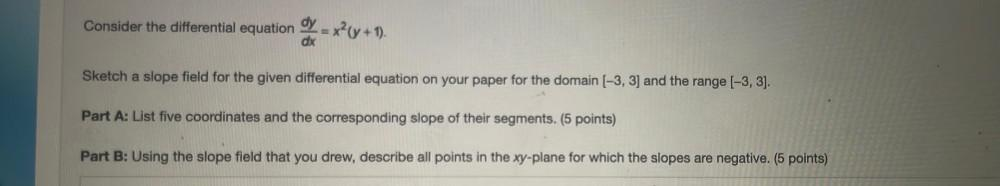Question:

# Consider the differential equation dy/dx=x^2(y + 1). Sketch a slope field for the given differential equation on your paper for the domain (-3, 3) and the range (-3, 3). A): List five coordinates andConsider the differential equation dy/dx=x^2(y + 1). Sketch a slope field for the given differential equation on your paper for the domain (-3, 3) and the range (-3, 3). A): List five coordinates and the corresponding slope of their segments. B): Using the slope field that you drew, describe all points in the xy-plane for which the slopes are negative.